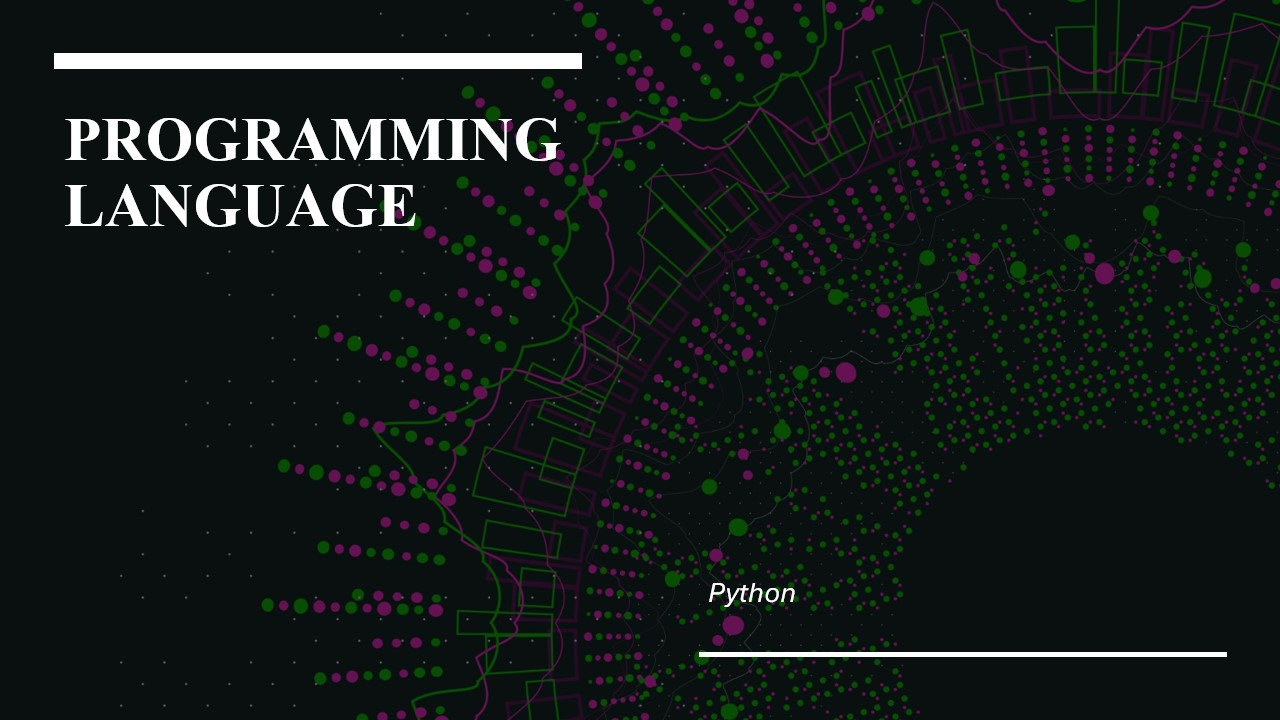/  Technology   /  How to print without newline or space in Python?## How to print without newline or space in Python?

Python’s print() function ends with a newline by default. So, if we use print(variable) then Python appends a newline to the output when displayed on the tty(teletypewriter A.K.A the terminal). Now, we would wonder how do we print two or more variables or statements without them going to a new line? How can we change the functioning of print()?

Newline or space

We can achieve this by changing the default values of the sep and end parameters of the print() function.

This solution works differently in different versions of Python. Let’s discuss each one of them.

#### Printing Without a Newline in Python 3.x

print was a reserved keyword that was like a special statement, until the Python version 2.x.It’s from the Python version 3.x, the print keyword has evolved into a function.

This print() function takes in the following parameters:

print(var1,var2,  ….sep,end)

var1,va2….: The values of these can be any of the data types like list, float, string, etc.

sep: This is called the separator which is used to divide the values given as arguments.

end: This argument by default is the ‘\n’ newline character. This is the reason why whenever the print() function is called, the cursor slides to the next line.

To print without a newline In Python 3.x, we  have to set the end argument as an empty string i.e. ‘ ‘.

Example:

```>>> print("I am a CS Student", "I am a CS Student")
I am a CS Student I am a CS Student
>>>```

Output:We want the two strings to print together. So here in the above example, Python by default gives the value of the argument sep, a blank space. Python also adds a newline character at the end, hence we can see that the interpreter prompt goes to the next line.

Example:

```>>> print ("I am a CS Student", "I am a CS Student", sep="; ", end="")
I am a CS Student; I am aCS Student>>>```

Output:In this example, if we can notice that two things have happened

The separator now includes a semicolon between the two strings. The interpreter prompt also appears on the same line as we have removed the default newline character.

#### Printing Without a Newline in Python 2.x

For the earlier versions of Python 3.x and the later versions of 2.6. We can import the print() function from the __future__ module. This overrides the existing print keyword with the print() function as shown below:

Example:

```>>> #In Python 2.6  and greater
>>> from __future__ import print_function
>>> print("I am a sentence", "I am also a sentence", sep="; ", end="")
I am a sentence; I am also a sentence>>>```

Output:This is how we can use Python version 3’s print() function in Python 2.x.

One can use these discussed strategies while printing the elements in the outputs of algorithms such as a binary tree or while printing the contents of a list next to each other.